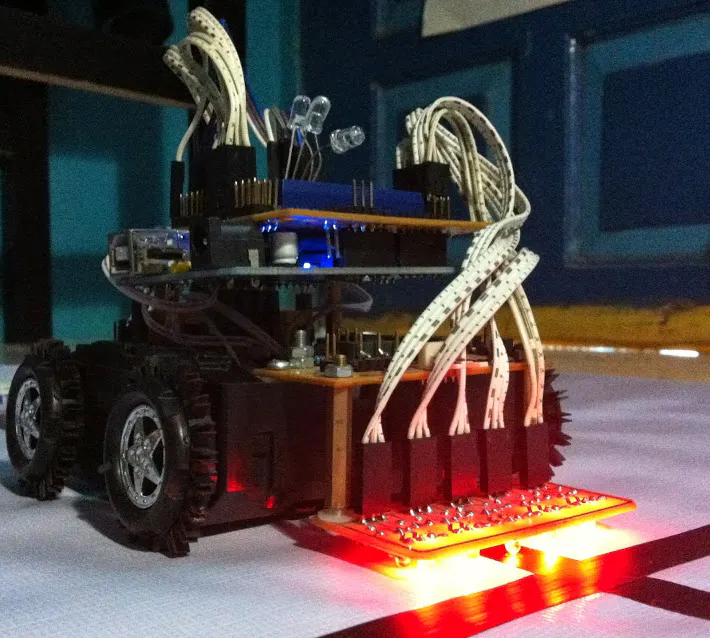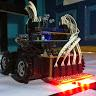Project showcase# Shortest Path of Line Maze - Curved and Zig-zag Track © GPL3+

Traverse all path and find the shortest path from starting point to finish.

• 5,852 views
• 21 respects

## Components and suppliesArduino UNO I got it installed on a line follower mobile robot, from Kokaro (Komunitas Kampung Robot) through Mr. Arief Andi Yudanarko from Pendidikan Elektronika Negeri Surabaya.
×1

## Apps and online services

### Final Project of Bachelor's Degree in Informatics Engineering

This project is about a line follower mobile robot that is used to find the shortest path from the starting point to the end of maze. The track is made of black tape on a white surface. In this project we used a white backdrop. The difference between the maze of this project and the previous one is that we have to make this mobile robot works on a track with curved and zig-zag track. It was for the final project of my bachelor's degree that finished in July of 2014.

A paper about this project has been published in 2018 Seventh ICT International Student Project Conference (ICT-ISPC).

Date of Conference: 11-13 July 2018

Date Added to IEEE Xplore: 08 November 2018

Publisher: IEEE

Conference Location: Nakhonpathom, Thailand, Thailand

## Code

##### Line Maze Solving - Curved and Zig-zag TrackArduino
Just copy and paste this to your Arduino IDE.

I am using right hand rule.

Sorry that's all for now (12 Aug 2017 5:38AM)
I don't have more time to update more of this now.

Someone asked about the "O" in "void choosepath()", then I realized that I have to update the "void shortpath()". (10 May 2019 10:38 MYT)
```// R. Joko Musridho
// Informatics Engineering – Faculty of Science and Technology
// UIN SUSKA RIAU
// Pekanbaru, Riau, Indonesia

// this was my Bachelor’s Degree final project (viva voce on July 22nd, 2014)

int sensor0 = A4; //leftmost sensor, pin A4
int sensor1 = A3;
int sensor2 = A2;
int sensor3 = A1;
int sensor4 = A0;// rightmost sensor, pin A0

int mata0; // mata means “eye”
int mata1;
int mata2;
int mata3;
int mata4;

int motorR = 4; //forward or reverse, pin 4
int onR = 5; //magnitude of the power for the motor
int onL = 6; //magnitude of the power for the motor
int motorL = 7; //forward or reverse, pin 7

int data;
unsigned char s; //array for sensors

int pathlength; //variable to record the total of path length
int readpath; //variable to call the path record
char path; //array for path record

int threshold = 990; //threshold between black/white

//led to show what the robot is recording (L/R/S/U)
int BLUEled = 13;
int GREENled = 12;
int YELLOWled = 11;

void strongright()
{
analogWrite(onL, 255);
digitalWrite(motorL, HIGH);
analogWrite(onR, 105);
digitalWrite(motorR, LOW);//to keep the robot on the line
}

void midright2()
{
analogWrite(onL, 255);
digitalWrite(motorL, HIGH);
analogWrite(onR, 105);
digitalWrite(motorR, HIGH);
}

void midright()
{
analogWrite(onL, 255);
digitalWrite(motorL, HIGH);
analogWrite(onR, 125);
digitalWrite(motorR, HIGH);
}

void softright()
{
analogWrite(onL, 255);
digitalWrite(motorL, HIGH);
analogWrite(onR, 225);
digitalWrite(motorR, HIGH);
}

void moveforward()
{
analogWrite(onL, 255);
digitalWrite(motorL, HIGH);
analogWrite(onR, 255);
digitalWrite(motorR, HIGH);
}

void softleft()
{
analogWrite(onL, 225);
digitalWrite(motorL, HIGH);
analogWrite(onR, 255);
digitalWrite(motorR, HIGH);
}

void midleft()
{
analogWrite(onL, 125);
digitalWrite(motorL, HIGH);
analogWrite(onR, 255);
digitalWrite(motorR, HIGH);
}

void midleft2()
{
analogWrite(onL, 105);
digitalWrite(motorL, HIGH);
analogWrite(onR, 255);
digitalWrite(motorR, HIGH);
}

void strongleft()
{
analogWrite(onL, 105);
digitalWrite(motorL, LOW);//to keep the robot on the line
analogWrite(onR, 255);
digitalWrite(motorR, HIGH);
}

void turnright()
{
analogWrite(onL, 255);
digitalWrite(motorL, HIGH);
analogWrite(onR, 255);
digitalWrite(motorR, LOW);
}

void turnleft()
{
analogWrite(onL, 255);
digitalWrite(motorL, LOW);
analogWrite(onR, 255);
digitalWrite(motorR, HIGH);
}

void righttillstraight()
{
turnright();
delay(500); //to be free from the line if there is a straight intersection (exit 2)
while (s==0)
{
}
}

void lefttillstraight()
{
turnleft();
delay(500); //to be free from the line if there is a straight intersection (exit 2)
while (s==0)
{
}
}

void turnaround()
{
lefttillstraight();
}

void stop()
{
analogWrite(onL, 0);
digitalWrite(motorL, LOW);
analogWrite(onR, 0);
digitalWrite(motorR, LOW);
}

void lilmoveforward()
{
moveforward();
delay(150); // adjust based on your batteries power, new one would be stronger than the drained-out one, of course
}

void ONforBLUEled()
{
digitalWrite (BLUEled, HIGH); delay(25);
digitalWrite (BLUEled, LOW);
}

void ONforGREENled()
{
digitalWrite (GREENled, HIGH); delay(25);
digitalWrite (GREENled, LOW);
}

void ONforYELLOWled()
{
digitalWrite (YELLOWled, HIGH); delay(25);
digitalWrite (YELLOWled, LOW);
}

{
//if declared outside, the sensor readings become “11111”

//converting to digital
if (mata0 < threshold)
{s = 1;}
else
{s = 0;}

if (mata1 < threshold)
{s = 1;}
else
{s = 0;}

if (mata2 < threshold)
{s = 1;}
else
{s = 0;}

if (mata3 < threshold)
{s = 1;}
else
{s = 0;}

if (mata4 < threshold)
{s = 1;}
else
{s = 0;}

//change the sensor readings into a series of binary number
data=(s*16)+(s*8)+(s*4)+(s*2)+(s*1);

//to display the sensor readings on serial monitor
Serial.print(s);
Serial.print("   ");
Serial.print(s);
Serial.print("   ");
Serial.print(s);
Serial.print("   ");
Serial.print(s);
Serial.print("   ");
Serial.print(s);
Serial.println(" ");
}

//Intersection condition -------------- 0b00abcde
void condition()
{
if (data==0b0000100)
{moveforward();}
else if (data==0b0000001)
{
strongright();
while (s==0)
{
strongright();
}
}
else if (data==0b0000011)
{midright2();}
else if (data==0b0000010)
{midright();}
else if (data==0b0000110)
{softright();}
else if (data==0b0001100)
{softleft();}
else if (data==0b0001000)
{midleft();}
else if (data==0b0011000)
{midleft2();}
else if (data==0b0010000)
{
strongleft();
while (s==0)
{
strongleft();
}
}
else //there is a right angle turn or intersection
{
{
turnaround();
path[pathlength]='U';pathlength++;//save U
ONforYELLOWled();
}
else if (data==0b0011111) //T, +, end of maze
{
lilmoveforward();
if (data==0b0000000)// T intersection
{
lilmoveforward(); //turning stabilizer
righttillstraight();
path[pathlength]='R';pathlength++;//save R
ONforGREENled();
}
else if (data==0b0011111)//end of maze
{
stop(); //stopping the robot
path[pathlength]='F';pathlength++;//save F

//sign for the end of maze
ONforBLUEled();delay(300);
ONforBLUEled();delay(300);
ONforYELLOWled();delay(300);
ONforYELLOWled();delay(300);
ONforGREENled();delay(300);
ONforGREENled();delay(300);
ONforBLUEled();ONforYELLOWled();ONforGREENled();

shortpath(); //calculate the shortest path
ONforBLUEled();ONforYELLOWled();ONforGREENled();

//sign to prepare the robot (put it back) on the starting position
ONforBLUEled();delay(300);
ONforYELLOWled();delay(1000);
ONforGREENled();delay(300);
ONforGREENled();delay(300);
ONforGREENled();delay(1000);

shortestpath(); //move through the shortest path
}
else //ada garis ke arah lurus 00100 dll (+ intersection)
{
lilmoveforward(); //turning stabilizer
righttillstraight();
path[pathlength]='R';pathlength++;//save R
ONforGREENled();
}
}
//straight or right junction
else if ((data==0b0000111)||(data==0b0001111))
{
lilmoveforward();lilmoveforward();
if (data==0b0000000)//right only
{
lilmoveforward();
righttillstraight();
}
else if (s==0)
{
lilmoveforward();
righttillstraight();
path[pathlength]='R';pathlength++;//save R
ONforGREENled();
}
}
//left or straight junction
else if ((data==0b0011100)||(data==0b0011110))
{
lilmoveforward();lilmoveforward();
if (data==0b0000000) //left only
{
lilmoveforward();
lefttillstraight();
}
else if (s==0) //there is a straight path
{
moveforward();
path[pathlength]='S';pathlength++;//save S
ONforBLUEled();
}
}
}
}

void shortpath() //calculate the shortest path
{
//because (..F) is the last and there is no U recorderd before F
int x = (pathlength-2);

while (x > 0)
{
if (path[x]=='U')
{
if (path[x-1]=='L' && path[x+1]=='L')
{path[x-1]='S';path[x]='O';path[x+1]='O';}
else if (path[x-1]=='L' && path[x+1]=='S')
{path[x-1]='R';path[x]='O';path[x+1]='O';}
else if (path[x-1]=='R' && path[x+1]=='R')
{path[x-1]='S';path[x]='O';path[x+1]='O';}
else if (path[x-1]=='R' && path[x+1]=='S')
{path[x-1]='L';path[x]='O';path[x+1]='O';}
else if (path[x-1]=='S' && path[x+1]=='L')
{path[x-1]='R';path[x]='O';path[x+1]='O';}
else if (path[x-1]=='S' && path[x+1]=='R')
{path[x-1]='L';path[x]='O';path[x+1]='O';}
else if (path[x-1]=='L' && path[x+1]=='R')
{
path[x-1]='U';path[x]='O';path[x+1]='O';

}
else if (path[x-1]=='R' && path[x+1]=='L')
{
path[x-1]='U';path[x]='O';path[x+1]='O';
if
}
else if (path[x-1]=='S' && path[x+1]=='S')
{
path[x-1]='U';path[x]='O';path[x+1]='O';

}
//---------------------------------------
x--;
}
else
{x--;}
}
}

void shortestpath()
{
if (data==0b0000100)
{moveforward();}
else if (data==0b0000001)
{
strongright();
while (s==0)
{
strongright();
}
}
else if (data==0b0000011)
{midright2();}
else if (data==0b0000010)
{midright();}
else if (data==0b0000110)
{softright();}
else if (data==0b0001100)
{softleft();}
else if (data==0b0001000)
{midleft();}
else if (data==0b0011000)
{midleft2();}
else if (data==0b0010000)
{
strongleft();
while (s==0)
{
strongleft();
}
}
else
//right or straight
if ((data==0b0000111)||(data==0b0001111))
{
lilmoveforward();
if (data==0b0000000)//right only
{
lilmoveforward();
righttillstraight();
}
else //there is a straight path
{
choosepath();
}
}
//left or straight
else if ((data==0b0011100)||(data==0b0011110))
{
lilmoveforward();
if (data==0b0000000) //left only
{
lilmoveforward();
lefttillstraight();
}
else //there is a straight path
{
choosepath();
}
}
//T, +, or END OF MAZE
else if  (data==0b0011111)
{
choosepath();
}
shortestpath();
}

void choosepath()//to get rid of the effect of “path[]==0” in the record
{
{
stop();finish();
}
{
ONforGREENled();
righttillstraight();
}
{
ONforGREENled();ONforBLUEled();
moveforward();delay(200);
}
{
ONforGREENled();ONforYELLOWled();
lefttillstraight();
}
{
}
shortestpath();
}

void finish()
{
ONforBLUEled();delay(100);
ONforYELLOWled();delay(100);
ONforGREENled();delay(100);
finish();
}

void setup()
{
Serial.begin(9600);
pinMode(motorR, OUTPUT);
pinMode(motorL, OUTPUT);
pinMode(onR, OUTPUT);
pinMode(onL, OUTPUT);
pinMode(BLUEled, OUTPUT);
pinMode(YELLOWled, OUTPUT);
pinMode(GREENled, OUTPUT);
analogReference(INTERNAL);
}

void loop()
{
condition();
}
```

#### Team MusridhoRJ YantoF YudanarkoAA TriD• 1 project
• 7 followers

August 13, 2017

#### Members who respect this project

See similar projects
you might like

• 15,492 views
• 57 respects

#### Arduino Marble Maze Labyrinth

Project tutorial by AhmedAzouz

• 14,398 views
• 46 respects

#### 2016 Halloween Laser Maze

Project showcase by Sam Horne

• 13,291 views
• 31 respects

#### Challenging Maze Game

Project showcase by gmussi

• 2,331 views
• 8 respects

#### Big Track Rover "ENHANCED EDITION" Obstacle Avoidance Bot

Project showcase by Sam

• 1,494 views
• 1 comment
• 3 respects

#### How to Make a Remote Controlled Robotic Hand with Arduino

Project showcase by Gabry295

• 46,232 views